# Test: Electronics & Communication- 1

## 25 Questions MCQ Test Basic Electronics Engineering for SSC JE (Technical) | Test: Electronics & Communication- 1

Description
Attempt Test: Electronics & Communication- 1 | 25 questions in 60 minutes | Mock test for Electrical Engineering (EE) preparation | Free important questions MCQ to study Basic Electronics Engineering for SSC JE (Technical) for Electrical Engineering (EE) Exam | Download free PDF with solutions
QUESTION: 1

Solution:
QUESTION: 2

Solution:
QUESTION: 3

###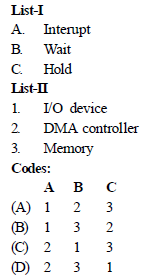Solution:
QUESTION: 4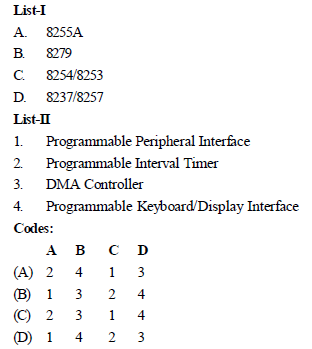Solution:
QUESTION: 5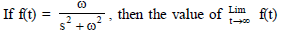Solution:
QUESTION: 6

The capacitance and loss angle of a given capacitor specimen are best measured by

Solution:
QUESTION: 7

Wagner's earth devices are used in ac bridge circuits to

Solution:
QUESTION: 8

The gauge factor is defined as

Solution:
QUESTION: 9

Hot wire anemometer is a device used to measure the

Solution:
QUESTION: 10

If the response of a system to an input does not depend on the future values of the input, then which one of the following is true for the system ?

Solution:
QUESTION: 11

The discrete time system described by y(n) = x(n)2 is

Solution:
QUESTION: 12

Effective Q of the equivalent electrical circuit of quartz crystal is of the order of

Solution:
QUESTION: 13

The converse of magnetostriction effect is

Solution:
QUESTION: 14

The phenomenon known as "Early Effect" in a bipolar transistor refers to a reduction of the effective base-width caused by

Solution:
QUESTION: 15

Which of the following are true for h-parameters of transistors ?

1. They are real numbers at audio frequencies

2. They are easy to measure

3. They vary widely with temperature

Select the correct answer using the code given below:

Solution:
QUESTION: 16

Which one of the following bridges will be used for the measurement of very low resistance ?

Solution:
QUESTION: 17

The X- and Y-inputs of a CRO are respectively V sin φt and -V sin φt . The resulting Lissajous pattern will be

Solution:
QUESTION: 18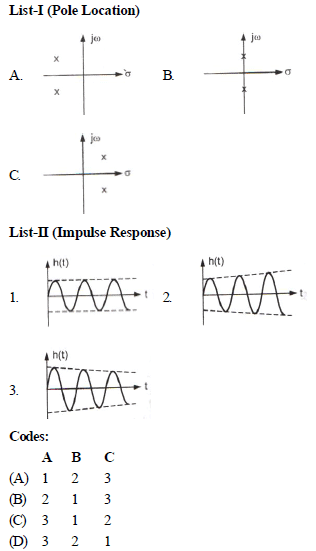Solution:
QUESTION: 19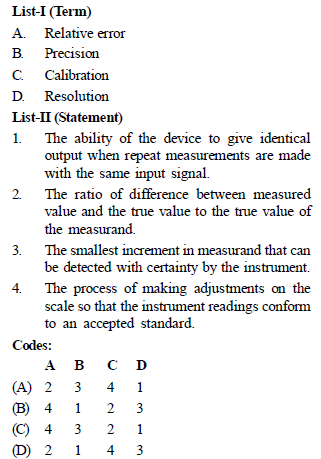Solution:
QUESTION: 20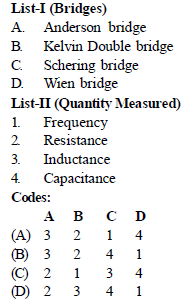Solution:
QUESTION: 21

In a bistable multivibrator, commutating capacitors are used to

Solution:
QUESTION: 22

The switching circuit given in the figure can be expressed in binary logic notation as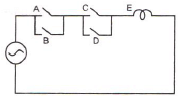Solution:
QUESTION: 23

Assuming that only logic inputs X and Y are available and their complements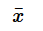and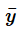are not available, the minimum number of two-input NAND gates required to implement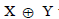would be

Solution:
QUESTION: 24

Which one of the following is not a property of root loci

Solution:
QUESTION: 25

For frequencies up to 1650 kHz, the transmitting antenna used is a

Solution:Use Code STAYHOME200 and get INR 200 additional OFF Use Coupon Code